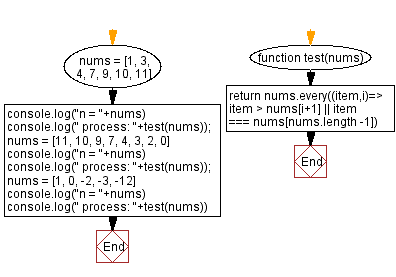# JavaScript: Check downward trend, array of integers

## JavaScript Math: Exercise-93 with Solution

Write a JavaScript program to check if an array of integers has a downward trend or not.

Test Data:
([1, 3, 4, 7, 9, 10, 11]) -> false
([11, 10, 9, 7, 4, 3, 2, 0]) -> true
([1, 0, -2, -3, -12]) -> true

Sample Solution:

HTML Code:

``````<!DOCTYPE html>
<html>
<meta charset="utf-8">
<title>JavaScript program to Check downward trend, array of integers</title>
<body>

</body>
</html>
```
```

JavaScript Code:

``````function test(nums) {
return nums.every((item,i)=> item > nums[i+1] || item === nums[nums.length -1])
}
nums = [1, 3, 4, 7, 9, 10, 11]
console.log("n = "+nums)
console.log(" process: "+test(nums));
nums = [11, 10, 9, 7, 4, 3, 2, 0]
console.log("n = "+nums)
console.log(" process: "+test(nums));
nums = [1, 0, -2, -3, -12]
console.log("n = "+nums)
console.log(" process: "+test(nums))
```
```

Sample Output:

```n = 1,3,4,7,9,10,11
process: false
n = 11,10,9,7,4,3,2,0
process: true
n = 1,0,-2,-3,-12
process: true
```

Flowchart:Live Demo:

See the Pen javascript-math-exercise-93 by w3resource (@w3resource) on CodePen.

Improve this sample solution and post your code through Disqus

What is the difficulty level of this exercise?

Test your Programming skills with w3resource's quiz.

﻿